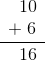Common Core: 2nd Grade Math : Read and Write Numbers to 1000 by Numerals, Number Names, and Expanded Form: CCSS.Math.Content.2.NBT.A.3

Example Questions

← Previous 1 3 4 5 6 7 8 9 35 36

Example Question #9 : Whole And Part

What number is one thousand?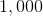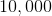Explanation:in word form is one thousand.

Example Question #10 : Whole And Part

What number is one hundred?Explanation:in word form is one hundred.

Example Question #1 : Identifying Numbers

What number is two hundred?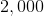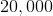Explanation:in word form is two hundred.

Example Question #2 : Identifying Numbers

What number is nine hundred?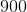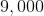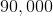Explanation:in word form is nine hundred.

Example Question #3 : Identifying Numbers

What number is forty-three?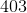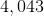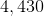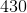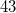Explanation:in word form is forty-three.

Example Question #4 : Identifying Numbers

What number is eight hundred four?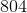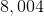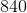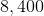Explanation:in word form is eight hundred forty-four.

Example Question #5 : Identifying Numbers

What number is ninety-six?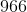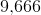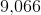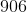Explanation:in word form is ninety-six.

Example Question #6 : Identifying Numbers

What number is nine hundred forty-eight?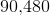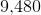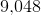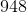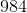Explanation:in word form is nine hundred forty-eight.

Example Question #1 : Read And Write Numbers To 1000 By Numerals, Number Names, And Expanded Form: Ccss.Math.Content.2.Nbt.A.3

The number below is written in expanded form. What is the number as a numeral?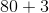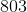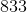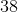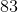Explanation:

When a number is in expanded form, you add the numbers together to find the numeral.

The numeral form of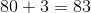.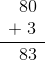Example Question #2 : Read And Write Numbers To 1000 By Numerals, Number Names, And Expanded Form: Ccss.Math.Content.2.Nbt.A.3

The number below is written in expanded form. What is the number as a numeral?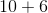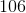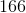The numeral form of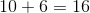.